# MCAT Physical : Boiling Point

## Example Questions

← Previous 1

### Example Question #1 : Boiling Point

The values for normal boiling and freezing points, along with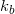and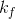values are given below for select solvents.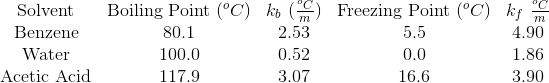A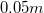solution of which of the following compositions would result in the greatest boiling point elevation?

Magnesium phosphide in benzene

Sodium chloride in acetic acid

Magnesium phosphide in acetic acid

Sodium chloride in benzene

Magnesium phosphide in acetic acid

Explanation:

Boiling point elevation depends on three variables: the boiling point elevation constant of the solvent, the van't Hoff factor of the solute, and the molality of the solution. In this question, molality is held constant.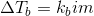First, calculate the van't Hoff for each compound.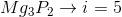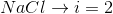Ultimately, we are looking for the greatest product of the boiling point elevation constant and van't Hoff factor (since molality is constant).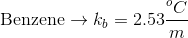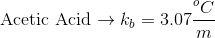Magnesium phosphide has the greater van't Hoff factor and acetic acid has the greater boiling point elevation constant. A solution of magnesium phosphide in acetic acid will thus have the greatest boiling point elevation.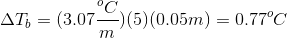### Example Question #2 : Boiling Point

The values for normal boiling and freezing points, along withandvalues are given below for select solvents.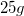of ammonium phosphate are dissolved in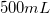of water. What is the boiling point of this solution at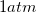?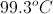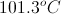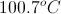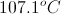Explanation:

We first need to find the boiling point elevation with the equation:Ammonium phosphate has an van't Hoff value of four; each molecule dissociates into four ions in solution. To calculate the molality, we need to find moles of solute per kilogram of solution.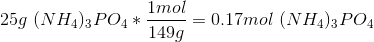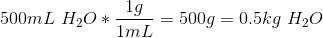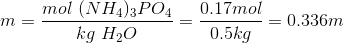Next, use the molality, van't Hoff factor, and boiling point elevation constant to solve for the increase in boiling point.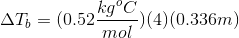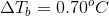Add this increase to the boiling point of pure water to find the boiling point of the solution.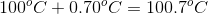### Example Question #3 : Boiling Point

Boiling point is the temperature a liquid needs to achieve in order to begin its transformation into a gaseous state. Campers and hikers who prepare food during their trips have to account for differences in atmospheric pressure as they ascend in elevation. During the ascent, the decrease in atmospheric pressure changes the temperature at which water boils.

Further complicating the matter is the observation that addition of a solute to a pure liquid also changes the boiling point. Raoult’s Law can be used to understand the changes in boiling point if a non-volatile solute is present, as expressed here.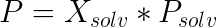In this law,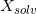is the mole fraction of the solvent,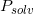is the vapor pressure of the pure solvent, andis the vapor pressure of the solution. When this vapor pressure is equal to the local atmospheric pressure, the solution boils.

Two campers are preparing food at an altitude of 13,000 feet on a mountain in Colorado. Which of the following is true as they boil a pot of water?

They will likely have to cook their food a shorter time than at sea level, since it takes less heat to make vapor pressure match atmospheric pressure

They will likely have to cook their food a shorter time than at sea level, since it takes more heat to make vapor pressure match atmospheric pressure

They will likely have to cook their food longer than at sea level, since it takes less heat to make vapor pressure match atmospheric pressure

If they add salt to the water, it will help speed the rate at which the water boils

They will likely have to cook their food longer than at sea level, since it takes more heat to make vapor pressure match atmospheric pressure

They will likely have to cook their food longer than at sea level, since it takes less heat to make vapor pressure match atmospheric pressure

Explanation:

The local atmospheric pressure at 13,000 feet is less than the pressure at sea level; therefore, it takes less heat to make the vapor pressure meet the local atmospheric pressure. Heat added to the system easily exits again as the water is converted to steam, leaving less heat in the water to cook the food. Food cooks more slowly as a result.

### Example Question #4 : Boiling Point

Boiling point is the temperature a liquid needs to achieve in order to begin its transformation into a gaseous state. Campers and hikers who prepare food during their trips have to account for differences in atmospheric pressure as they ascend in elevation. During the ascent, the decrease in atmospheric pressure changes the temperature at which water boils.

Further complicating the matter is the observation that addition of a solute to a pure liquid also changes the boiling point. Raoult’s Law can be used to understand the changes in boiling point if a non-volatile solute is present, as expressed here.In this law,is the mole fraction of the solvent,is the vapor pressure of the pure solvent, andis the vapor pressure of the solution. When this vapor pressure is equal to the local atmospheric pressure, the solution boils.

The change in boiling point with addition of a solute is a colligative property of a solution. Which of the following are also examples of colligative properties?

I. Vapor pressure reduction

II. Color emission with dissolution of a solute

III. Osmotic pressure

I and II, only

III, only

I and III, only

I, II, and III

II and III, only

I and III, only

Explanation:

Colligative properties are defined as properties that depend entirely upon the ratio of the number of solute particles to the number of solvent particles. Only osmotic pressure and vapor pressure depression are examples of such phenomena. While color emission is a property of a solution, it depends on the chemical species involved, and not the number of particles.

### Example Question #5 : Boiling Point

Suppose two containers each contain the same amount of solvent. 2m NaCl solution is added to the first container, and a mystery solution is added to the second container. Upon heating the flasks, it is determined that the second container has a higher boiling point than the first container. Assume the solutions are ideal.

Based on the above information, which of the following compounds could have been added to container 2?

2m CaF2

3m C6H12O6

1m MgCl2

1m NaCl

2m CaF2

Explanation:

Based on the equation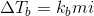, we see that there are two factors that differ between the containers and can affect the elevation of the boiling point: molality and the van't Hoff factor ().

The sodium choride added to container 1 has a molality of 2, as well as a van't Hoff factor of 2. As a result, we are looking for a compound that has a larger combination of these two factors, which would cause a higher boiling point. 2m CaF2 has a molality of 2 and a van't Hoff factor of 3. Since this combination of factors in container 2 would be higher than the combination in container 1, we can conclude that this was the mystery compound added to the container with the higher boiling point.

Note that C6H12O6 is the formula for glucose, and will not ionize in solution.

### Example Question #6 : Boiling Point

The equation for boiling point elevation is written as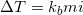.

The "" value in the equation is referred to as the van't Hoff factor, and is the number of particles that the solute is expected to dissociate into once in solution. In ideal solutions, ions will separate entirely, and the van't Hoff factor will be the expected value; however, nonideal solutions can have ion pairing take place, where ions do not separate entirely. As a result, the observed van't Hoff factor will be slightly less than the expected van't Hoff factor.

If a solution has ion pairing taking place, which statement is true?

The actual boiling point elevation will be lower than the theoretical boiling point elevation.

The vapor pressure of the solution will be lower than predicted.

The molality of the solution will decrease.

The solute will not raise the boiling point of the solution.

The actual boiling point elevation will be lower than the theoretical boiling point elevation.

Explanation:

If there is ion pairing taking place in a solution, the van't Hoff factor will be slightly lower than predicted. As a result, the boiling point will not be as elevated as it would be if all of the ions were separated from each other. This conclusion can be draw from the given equation,. The number of particles in the solution does not affect the molality.

### Example Question #7 : Boiling Point

Which of the following aqueous solutions will have the highest boiling point?

1m MgCl2

1m NaF

2m MgCl2

2m NaCl

2m MgCl2

Explanation:

In order to answer this problem, consider the equation for boiling point elevation:.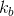is a specific constant for the boiling substance, so it will not change between the solutions (they are all aqueous). Molality is designated as "m", and a high molality will result in a higher boiling point, however, the value we want to look at for this problem is, which is also known as the van't Hoff factor. The van't Hoff factor is the number of particles that a single solute will dissociate into when added to a solution. MgClwill dissociate into three particles: 1 Mg2+ cation and 2 Cl- anions. Since 2m of MgCl2 has the highest molality as well as the largest van't Hoff factor out of the options, it will result in the highest boiling point.

### Example Question #8 : Boiling Point

Which solution will have a higher boiling point?

Solution 1: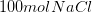in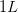water

Solution 2: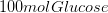inwater

Solution 1

Solution 1 and 2 will have the same boiling point

The answer cannot be determined from the information given

Solution 2

Solution 1

Explanation:

Adding solute to water will result in boiling point elevation due to the presence of more molecules. Change in temperature is given by the relation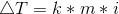, whereis a constant for the solvent,is the solution molality, andis the van't Hoff factor. In this example, the molalities are equal.

Since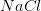dissociates into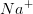and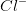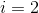, representing the two ions derived from each molecule. For glucose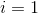, as the molecule does not dissociate. Solution 1 will have a higher elevation in temperature due to the greater number of ions in solution.

### Example Question #9 : Boiling Point

Which of the following compounds will create the greatest increase in boiling point when added to an aqueous solution?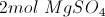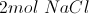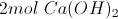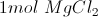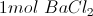Explanation:

Colligative properties are dependent only on the number of particles in a solution, and not their identity. Some examples of colligative properties are vapor pressure, boiling point, freezing point, and osmotic pressure.

There is a direct relationship between the boiling point elevation and the number of particles present in a solution. The more particles that are present in solution, the higher the boiling point elevation.

We are looking for the compound that will create the greatest number of ions when dissolved in solution.

Sodium chloride and magnesium sulfate will produce two ions per mole.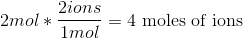Magnesium chloride and barium chloride will produce three ions per mole.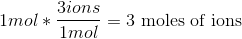Calcium hydroxide will also produce three ions per mole, but we are given two moles instead of one.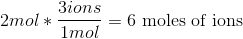Calcium hydroxide will produce the greatest number of ions, thus creating the greatest increase in boiling point elevation.

### Example Question #10 : Boiling Point

Each of the following solutions is added to equal amounts of water. Which solution will result in the greatest amount of boiling point elevation?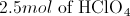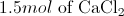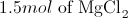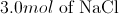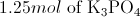Explanation:

Boiling point elevation is a colligative property, meaning that it depends on the relative number of solute particles in solution. The answer choice with the largest number of moles of particles will show the greatest boiling point elevation. The equation for boiling point elevation is:Molality is equal to moles of solute per kilogram of solvent, meaning that it will be proportional to the moles of solute added. Each solute is added to equal amounts of water, allowing us to keep this value constant. Similarly,will be constant for all of the solutions. Overall, boiling point elevation will be proportional to the moles of solute multiplied by the van't Hoff factor.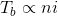Using this proportion, we can find the solute that will most impact the boiling point of water.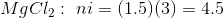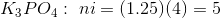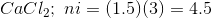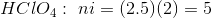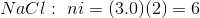Since sodium chloride results in the greatest moles of ions in solution, it will yield the greatest boiling point elevation.

← Previous 1